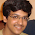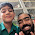May 26, 2011

Coloured Run of Cards

Problem:
There are 26 black(B) and 26 red(R) cards in a standard deck. A run is a maximum block of consecutive cards of the same color. For example, a sequence RRRRBBBRBRB of only 11 cards has
6 runs; namely, RRRR, BBB, R, B, R, B.

Find the expected number of runs in a shuffled deck of cards.

Update (29-05-2011):
Solution:
Posted by Siddhant Agarwal (EE IITB 2011 Alumnus) in comments!
Select the text between * to see the solution.
* Let us have n red cards and n black cards. Consider any sequence X_1,X_2..X_2n. Now define
Y_1 = 1,
Y_i = 1 if X_i and X_(i-1) are of diff colours
Y_i = 0 otherwise.

Clearly we have to find E[Y_1 + ..+Y_2n]
E[Y_1] = 1 by def.
E[Y_i] = E[Y_j] for all i,j>=2
by symmetry.

Also E[Y_i] = $2*(\binom{2n-2}{n-1})/($\binom{2n}{n})

so ans = 1+(2n-1)*E[Y_i] = n+1 *

1.I have used quite a similar approach to this one as the previous one (Bad Bars problem).

View the Math part using http://www.codecogs.com/latex/eqneditor.php

=== WARNING : SPOILER AHEAD ===

What we need to count is the number of runs cumulative over all possible permutations.
One way is to add the number of runs in each permutation.
Alternate way is to consider a particular run and count the number of permutations in which this particular run occurs; and then sum over all runs.

For example, suppose consider a run : RRRRR

This run occurs in one of the three places :
-- to left end
-- to the right end
-- somewhere in between

Each such run is surrounded by B on both sides (one side incase it is at the end).

Thus, the number of possible permutations in which this run occurs :
-- left end : $\binom{52-5-1}{25}$
-- right end : \binom{52-5-1}{25}
-- somewhere in between : (50-5+1)*\binom{52-5-2}{24}

Thus, summing over all possible runs :
2*\sum\limits_{x=1}^{n} \left[2\binom{2n-x-1}{n-1} + (n-x+1)\binom{2n-x-2}{n-2}\right]\\
= 4\sum\limits_{x=1}^{n} \binom{2n-x-1}{n-1} + (n+1)\sum\limits_{x=1}^{n}\binom{2n-x-2}{n-2} - \sum\limits_{x=1}^{n}x\binom{2n-x-2}{n-2} \\
= 4\binom{2n-1}{n} + (n+1)\binom{2n-2}{n-1} - \sum\limits_{y=0}^{n-1} \binom{n-1+y}{n-1}\\
= 4\binom{2n-1}{n} + (n+1)\binom{2n-2}{n-1} - \binom{2n-1}{n}\\
= 3\binom{2n-1}{n} + (n+1)\binom{2n-2}{n-1}

Thus, the expected value = \frac{3\binom{2n-1}{n} + (n+1)\binom{2n-2}{n-1}}{\binom{2n}{n}}

PS :
I have used some identities implicitly. I list them down here :
\sum\limits_{x=1}^{m} \binom{n+m-x}{n} = \binom{n+m}{n+1}

Using the above, you can easily derive :
\sum\limits_{x=1}^{m} x\binom{n+m-x}{n} = \binom{n+m+1}{n+2}

Question: Can you give an combinatorial argument for the last one?

2.Let us have n red cards and n black cards. Consider any sequence X_1,X_2..X_2n. Now define
Y_1 = 1,
Y_i = 1 if X_i and X_(i-1) are of diff colours
Y_i = 0 otherwise.

Clearly we have to find E[Y_1 + ..+Y_2n]
E[Y_1] = 1 by def.
E[Y_i] = E[Y_j] for all i,j>=2
by symmetry.

Also E[Y_i] = $2*(\binom{2n-2}{n-1})/($\binom{2n}{n})

so ans = 1+(2n-1)*E[Y_i] = n+1

3.@Siddhant.. Awesome. Thanks.

4.Yes.. There were a couple of calculation mistakes.

1) The number of runs of length 'x' of a particular colour that occur in between is (2n-x-1), instead of (n-x+1).
2) While expanding the bracket from first step to second step, I forgot to take the 2 inside.. :-/

Full derivation represented :
2*\sum\limits_{x=1}^{n} \left[2\binom{2n-x-1}{n-1} + (2n-x-1)\binom{2n-x-2}{n-2}\right]\\
= 4\sum\limits_{x=1}^{n} \binom{2n-x-1}{n-1} + 2(2n-1)\sum\limits_{x=1}^{n}\binom{2n-x-2}{n-2} - 2\sum\limits_{x=1}^{n}x\binom{2n-x-2}{n-2} \\
= 4\binom{2n-1}{n} + 2(2n-1)\binom{2n-2}{n-1} - 2\sum\limits_{y=0}^{n-1} \binom{n-1+y}{n-1}\\
= 4\binom{2n-1}{n} + 2(2n-1)\binom{2n-2}{n-1} - 2\binom{2n-1}{n}\\
= 2\binom{2n-1}{n} + 2(2n-1)\binom{2n-2}{n-1}\\
= 2\binom{2n-1}{n} + 2n\binom{2n-1}{n}\\
= 2(n+1)\binom{2n-1}{n}

So finally it gives expected value
= \frac{2(n+1)\binom{2n-1}{n}}{\binom{2n}{n}}
= n+1

PS: Awesome answer by Siddhant! :)

5.Thanks Pritish. Nice solution. Your solution is less aha based. :)

6.First let us calculate prob for having n runs when N is 26.
say n is even.

No.of.ways_n_even=2*[binom(N-1,(n-2)/2))]^2;
No.of.ways_n_odd=2*[binom(N-1,(n-1)/2))]*[binom(N-1,(n-3)/2))];
prob(n) = no.of.ways/ binom(2*N,N);

Exp.num.of.runs=sigma(n*prob(n));

7.8.Number of ways in which we can have 52 runs is same as number of ways in which we can have 2 runs. Similar result holds for 52-k and k+2. This can be explained using the following construction :
Suppose wlog we always start with red (this will not affect the expectation)
Then if we have 2k runs, then we need to place k "bars" between 26 red ones (with 1 bar necessarily at the end) and similarly for the black ones.
This can be done in (expression given by Avinash)
Similarly for 2k+1 bars, we need k+1 red bars and k black bars to be placed between 26 red and 26 black cards respectively ( This seperation by bars gives a unique representation for each sequence starting with red).
And now it is easy to see that 52-k and k+2 have the same number of arrangements possible, and thus the distribution is symmetric about 52+2/2 = 27.

9.Lets say we have even number of runs..That implies a run of rbrb...rbrb OR brbr...br where a b or a r consists of a set of Black or Red cards respectively.

N=26.
say n - even number of runs.
Then n/2 number of Red sets and n/2 number of Black sets.
Number of ways we can select n/2 Red sets from N cards is binom(N,n/2-1). and similarly for black sets.
Total number of ways arranging these n/2 + n/2 sets is 2*binom((N-1),n/2-1)*binom(N-1,n/2-1).

when n is odd
for the combin rbrb...r
(n+1)/2 red sets and (n-1)/2 black sets.
NUmber of ways of selecting (n+1)/2sets from N is binom(N-1,(n-1)/2) AND
selecting (n-1)/2 sets from N balls is binom(N-1,(n-3)/2).

Total ways for n odd hence is
2*binom(N-1,(n-1)/2)*binom(N-1,(n-3)/2)..

Average value is
sigma(n*no_of_ways/total_ways) where no of ways equals above expressions depending on n odd or even.
This is evaluating to N+1 for various N.(wrote a prog and verified)Lazy to simplify that expression and dont even know if it simplifies or not :D

Eg..
N=2;
Tot ways= 4c2=6.
for n=2 2*C(1,0)*C(1,0)=2=>1/3
for n=3 2*C(1,1)*C(1,0)=2=> 1/3
n=4 P =1/3
So avge is 3.

10.@Gautam.. Solution looks correct. Thanks.

@avinash.. Approach looks correct. But no points if its not solvable :P

11.@sid : Can you please elaborate on how you got the E[y_i]?

I got the same answer but on different idea.
I think E[Y_i]=2*(1/2)*((n)/(2n-1))
where n/2n-1 is just the ratio for different colour conditioned on first colour.

12.I have calculated E[Y_2]. Y_2 = 1 iff 1st card and 2nd card are of different colours. The prob that first two cards are different is (by simply calculating total possibilites)

$2*(\binom{2n-2}{n-1})/($\binom{2n}{n})

Hence the formula. It is clear that this is infact equal to (n/(2n-1)). Hence ur soln is also correct.

13.I think this problem can also be solved similar to one other problem on the blog
Here, we can form 51 random variables
X1, X2, ..., X51
Xi is the indicator random variable which is 1 if color of X(i+1) is different than X(i)
So, if X is X1 + X2 + X3 + ... + X51
E(X) = E(X1)+E(X2) + ... + E(X51)
i.e. Summation of Pis
E(Xi) = (26*26+26*26)/(26*26+26*26+26*25+26*25)
14.